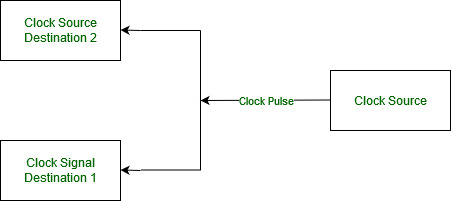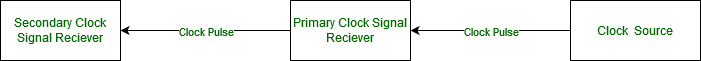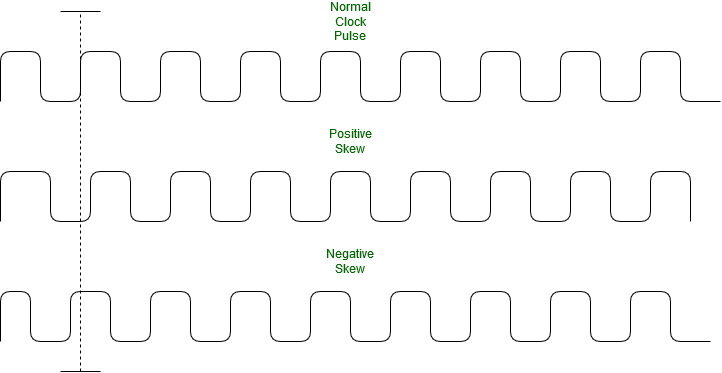# Clock Skew in synchronous digital circuit systems

• Difficulty Level : Medium
• Last Updated : 17 Jan, 2022

In Synchronous circuits where all the logic elements share the same clock signal, it becomes imperative to design these elements as close to the clock source as possible because a system-on-chip, FPGA, CPLD contain Billions of transistors. Even though these distances are minute due to their sheer number there is a propagation delay which leads to the clock signal arriving at different parts of the chip at different times. This is called Clock Skew.

In Digital Circuit Design a ” Sequentially Adjacent ” circuit is one where if a pulse emitted from a common source is supposed to arrive at the same time. Using this definition we can write a mathematical expression for clock skew asTa(Time of arrival of clock pulse at component a)
Tb(Time of arrival of clock pulse at component b)

Then,

`Clock skew Ts = Ta - Tb `

Factors causing Clock Skew :

• Interconnect Length

• Temperature Variations

• Capacitive Coupling

• Material Imperfections

• Differences in input capacitance on the clock inputs

Types of Clock Skew :

• Positive Skew –
This occurs when the receiving register receives the clock pulse later than it is required.

• Negative Skew –
This occurs when the receiving register receives the clock pulse earlier than required.Types of Clock Skews

My Personal Notes arrow_drop_up